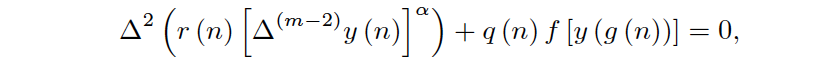##### Solutions to the Equations 2w – 3n ± 1=0 with w and n Positive Integers

Alain Jaeckel, Jean-François Palierne, Jean Dayantis

Journal of Advances in Mathematics and Computer Science, Page 1-6
DOI: 10.9734/BJMCS/2015/19252

We show that the equations 2w – 3n ± 1 = 0, where w and n are positive integers, have no other solutions than (w,n) = (1,0), (1,1), (2,1) and (3,2)1.

##### Computational Analysis for the Dynamical System Associated to an Access Control Structure

Adela Ionescu, Daniela Coman, Sonia Degeratu

Journal of Advances in Mathematics and Computer Science, Page 1-13
DOI: 10.9734/BJMCS/2015/19280

This paper presents some analytical considerations regarding the dynamical behavior of an access control structure, based on the mathematical model associated to this structure. This structure type is largely analyzed in the literature. A modern approach of this structure based on SMA (shape memory alloy) is taken into account, because of some particular advantages: unique characteristics (superelastic effect, as well as the single and double shape memory effects), damping capacity of noise and vibration, resistance to fatigue, diversification of the control and command possibilities.

The basic aim is the qualitative analysis of the mathematical model associated to this structure. Namely, the dynamic system associated to the variation of the angle q describing the position of the access control structure is analyzed from the influence of parameters standpoint. The MAPLE11 soft is used in order to evaluate the behavior of the equation solution with respect to the parameters variation.

This analysis produces a data collection which is useful both for further developing a fuzzy logic controller for the active control of this access structure and for further refinements of the mathematical model associated to this structure type.

##### Enhanced Ray Tracing Algorithm for Depth Image Generation

Hanan Ahmed, Howida A. Shedeed, Doaa Hegazy

Journal of Advances in Mathematics and Computer Science, Page 1-11
DOI: 10.9734/BJMCS/2015/19879

Ray tracing is a method to convert 3D image to high quality 2D realistic image. In traditional Ray tracing technique generating an image is an expensive process due to the large number of transmitted rays and the intersection tests of these rays with the scene primitives. This paper introduces an enhanced ray tracing (Enhanced RT) algorithm. In the proposed algorithm, merge sort algorithm is used to order triangles according to the minimum x coordinate. Then Binary Search algorithm is used to find the end index of the first triangle that has minimum x coordinate greater than the pixel x coordinate. This search limits the subset of the triangles that may intersect the ray, and hence, reduces the intersection calculation time. Experimental results show that the proposed algorithm decreased the execution time by 99.8% than the traditional ray tracing algorithm with high quality for the produced depth images for a standard Benchmark models. The implementation was done on an ordinary hardware without need to use the highly expensive parallel architecture hardware (as GPUs or Clusters) as in the other research in the same application. The proposed algorithm also achieved the highest successful hit rate in comparing to the most recent ray tracing algorithms.

##### Quick Techniques for Template Matching by Normalized Cross-Correlation Method

M. I. Khalil, Ahmed Ibrahim

Journal of Advances in Mathematics and Computer Science, Page 1-9
DOI: 10.9734/BJMCS/2015/16461

Object recognition is one of the fundamental challenges in signal processing, image processing and computer vision, where the goal is to identify and localize the extent of object instances within an image. A novel approach for performing the matching by normalized cross-correlation method in minimum time is introduced. The template matching by correlation is performed between template w and the image f where the template’s position is to be determined in the image. The computing process of correlation coefficient is analyzed and resolved into minute parts or units. These minute units are computed one time only before embedding them in larger blocks and stored in sum tables. The larger blocks are computed in recursive manner, using the sum tables, by adding and/or subtracting minute units from the original block instead of computing them from scratch. Moreover, this technique has been more developed by performing the cross-correlation on the odd or even signal’s samples only. The new approach, in its final form, has reduced the cross-correlation calculation time by 90%-94% depending on the image’s and template’s sizes.

##### Oscillation Criteria for Higher Order Nonlinear Functional Difference Equations

S. Kaleeswari, B. Selvaraj

Journal of Advances in Mathematics and Computer Science, Page 1-8
DOI: 10.9734/BJMCS/2015/20271

In this paper some criteria for the oscillation of high order functional difference equation of the formwhereis discussed. Examples are given to illustrate the results.

##### A 2-Step Four-Point Hybrid Linear Multistep Method for Solving Second Order Ordinary Differential Equations Using Taylor’s Series Approach

D. O. Awoyemi, O. O. Olanegan, O. B. Akinduko

Journal of Advances in Mathematics and Computer Science, Page 1-13
DOI: 10.9734/BJMCS/2015/5964

This paper considers the development of a 2-step four-point  continuous hybrid method for the direct solution of initial value problem (IVPs) of second order ordinary differential equations using the method of interpolation of the power series approximate solution and collocation of the differential system to develop our scheme. Taylor’s series approximation is used to analyze and implement yn+i i = 1...n-1  at xn+1, j = 0(1)2.  The method is found to be consistent and zero-stable.  Numerical results show a superior accuracy compared to existing methods.

##### Ranking Fuzzy Numbers by Using Dominance Based Approach

R. Suthakaran

Journal of Advances in Mathematics and Computer Science, Page 1-6
DOI: 10.9734/BJMCS/2015/20110

The most commonly used approach for ranking fuzzy numbers is ranking indices based on the centroids of fuzzy numbers. In this paper, we introduced a new ranking method which is based on multi-index such as relative dominance, priority weight and decision-makers preference. In addition to this we determined the feasibility and validity of this method by providing numerical examples.